##### Python All-in-One For Dummies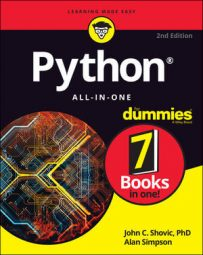The simplest data collection in Python is a list. A list is any list of data items, separated by commas, inside square brackets. Typically, you assign a name to the Python list using an = sign, just as you would with variables. If the list contains numbers, then don't use quotation marks around them. For example, here is a list of test scores:
`scores = [88, 92, 78, 90, 98, 84]`
If the list contains strings then, as always, those strings should be enclosed in single or double quotation marks, as in this example:

To display the contents of a list on the screen, you can print it just as you would print any regular variable. For example, executing `print(students)` in your code after defining that list shows this on the screen.

`['Mark', 'Amber', 'Todd', 'Anita', 'Sandy']`
This may not be exactly what you had in mind. But don’t worry, Python offers lots of great ways to access data in lists and display it however you like.

## Referencing Python list items by position

Each item in a list has a position number, starting with zero, even though you don’t see any numbers. You can refer to any item in the list by its number using the name for the list followed by a number in square brackets. In other words, use this syntax:
`<em>listname</em>[<em>x</em>]Replace <em><code>listname</code></em> with the name of the list you're accessing and replace <em><code>x</code></em> with the position number of item you want. Remember, the first item is always number zero, not one. For example, in the first line below, I define a list named <code>students</code>, and then print item number zero from that list. The result, when executing the code, is that the name <code>Mark</code> is displayed.`
```students = ["Mark", "Amber", "Todd", "Anita", "Sandy"]
print(students)
Mark```

When reading access list items, professionals use the word sub before the number. For example, students would be spoken as students sub zero.

This next example shows a list named `scores`. The `print()` function prints the position number of the last score in the list, which is 4 (because the first one is always zero).

```scores = [88, 92, 78, 90, 84]
print(scores)
84```
If you try to access a list item that doesn't exist, you get an “index out of range” error. The index part is a reference to the number inside the square brackets. For example, the image below shows a little experiment in a Jupyter notebook where a list of scores was created and then the printing of `score` was attempted.

It failed and generated an error because there is no `scores`. There's only `scores`, `scores`, `scores`, `scores`, and `scores` because the counting always starts at zero with the first one on the list.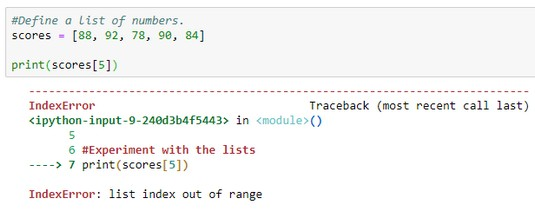Index out of range error because there is no scores.

## Looping through a Python list

To access each item in a list, just use a for loop with this syntax:
`for <em>x</em> in <em>list</em>:`
Replace >x with a variable name of your choosing. Replace `list` with the name of the list. An easy way to make the code readable is to always use a plural for the list name (such as `students`, `scores`). Then you can use the singular name (`student`, `score`) for the variable name. You don't need to use subscript numbers (numbers in square brackets) with this approach either. For example, the following code prints each score in the scores list:
```for score in scores:
print(score)```
Remember to always indent the code that’s to be executed within the loop. This image shows a more complete example where you can see the result of running the code in a Jupyter notebook.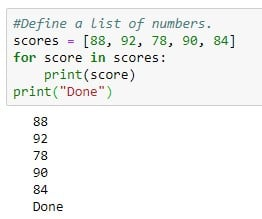Looping through a list.

## Seeing whether a Python list contains an item

If you want your code to check the contents of a list to see whether it already contains some item, use `in <em>listname</em>` in an `if` statement or a variable assignment.

For example, the code in the image below creates a list of names. Then, two variables store the results of searching the list for the names Anita and Bob. Printing the contents of each variable shows True for the one where the name (Anita) is in the list. The test to see whether Bob is in the list proves False.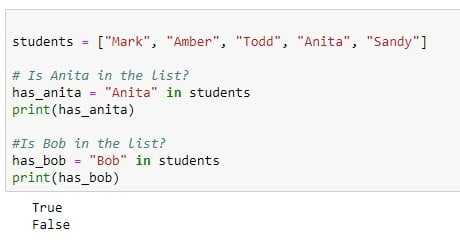Seeing whether an item is in a list.

## Getting the length of a Python list

To determine how many items are in a list, use the `len()` function (short for length). Put the name of the list inside the parentheses. For example, type the following code into a Jupyter notebook or Python prompt or whatever:
```students = ["Mark", "Amber", "Todd", "Anita", "Sandy"]
print(len(students))```
Running that code produces this output:
`5`
There are indeed five items in the list, though the last one is always one less than the number because Python starts counting at zero. So the last one, Sandy, actually refers to students and not students.

## Appending an item to the end of a Python list

When you want your Python code to add a new item to the end of a list, use the `.append()` method with the value you want to add inside the parentheses. You can use either a variable name or a literal value inside the quotation marks.

For instance, in the following image the line that reads `students.append("Goober")` adds the name Goober to the list. The line that reads `students.append(new_student)` adds whatever name is stored in the variable named `new_student` to the list. The `.append()` method always adds to the end of the list. So when you print the list you see those two new names at the end.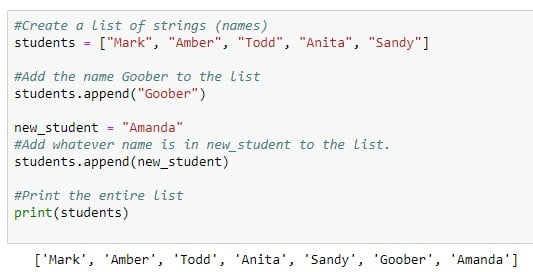Appending two new names to the end of the list.

You can use a test to see whether an item is in a list and then append it only when the item isn't already there. For example, the code below won’t add the name Amber to the list because that name is already in the list:

```student_name = "Amanda"#Add student_name but only if not already in the list.
if student_name in students:
print (student_name + " already in the list")
else:
students.append(student_name)
print (student_name + " added to the list")```

## Inserting an item into a Python list

Although the `append()` method allows you to add an item to the end of a list, the `insert()` method allows you to add an item to the list in any position. The syntax for `insert()` is
`<em>listname</em>.insert(<em>position</em>, <em>item</em>)`
Replace listname with the name of the list, position with the position at which you want to insert the item (for example, 0 to make it the first item, 1 to make it the second item, and so forth). Replace item with the value, or the name of a variable that contains the value, that you want to put into the list.

For example, the following code makes Lupe the first item in the list:

```#Create a list of strings (names).
students = ["Mark", "Amber", "Todd", "Anita", "Sandy"]student_name = "Lupe"
# Add student name to front of the list.
students.insert(0,student_name)#Show me the new list.
print(students)```
If you run the code, `print(students)` will show the list after the new name has been inserted, as follows:
`['Lupe', 'Mark', 'Amber', 'Todd', 'Anita', 'Sandy']`

## Changing an item in a Python list

You can change an item in a list using the = assignment operator (check out these common Python operators) just like you do with variables. Just make sure you include the index number in square brackets of the item you want to change. The syntax is:

listname[index]=newvalue

Replace `listname` with the name of the list; replace `index` with the subscript (index number) of the item you want to change; and replace `newvalue` with whatever you want to put in the list item. For example, take a look at this code:

```#Create a list of strings (names).
students = ["Mark", "Amber", "Todd", "Anita", "Sandy"]
students = "Hobart"
print(students)```
When you run this code, the output is as follows, because Anita's name has been changed to Hobart.
`['Mark', 'Amber', 'Todd', 'Hobart', 'Sandy']`

## Combining Python lists

If you have two lists that you want to combine into a single list, use the `extend()` function with the syntax:
`<em>original_list</em>.extend(<em>additional_items_list</em>)`
In your code, replace original_list with the name of the list to which you’ll be adding new list items. Replace additional_items_list with the name of the list that contains the items you want to add to the first list. Here is a simple example using lists named `list1` and `list2`. After executing `list1.extend(list2)`, the first list contains the items from both lists, as you can see in the output of the `print()` statement at the end.
```# Create two lists of Names.
list1 = ["Zara", "Lupe", "Hong", "Alberto", "Jake"]
list2 = ["Huey", "Dewey", "Louie", "Nader", "Bubba"]# Add list2 names to list1.
list1.extend(list2)# Print list 1.
print(list1)['Zara', 'Lupe', 'Hong', 'Alberto', 'Jake', 'Huey', 'Dewey', 'Louie', 'Nader', 'Bubba']```
Easy Parcheesi, no?

## Removing Python list items

Python offers a `remove()` method so you can remove any value from the list. If the item is in the list multiple times, only the first occurrence is removed. For example, the following code shows a list of letters with the letter C repeated a few times. Then the code uses `letters.remove("C")` to remove the letter C from the list:
```# Remove "C" from the list.
letters.remove("C")#Show me the new list.
print(letters)```
When you actually execute this code and then print the list, you'll see that only the first letter C has been removed:
`['A', 'B', 'D', 'C', 'E', 'C']`
If you need to remove all of an item, you can use a `while` loop to repeat the `.remove` as long as the item still remains in the list. For example, this code repeats the .remove as long as the “C” is still in the list.
```#Create a list of strings.
letters = ["A", "B", "C", "D", "C", "E", "C"]```
If you want to remove an item based on its position in the list, use `pop()` with an index number rather than `remove()` with a value. If you want to remove the last item from the list, use `pop()` without an index number.

For example, the following code creates a list, one line removes the first item (0), and another removes the last item `(pop()` with nothing in the parentheses). Printing the list shows those two items have been removed:

```#Create a list of strings.
letters = ["A", "B", "C", "D", "E", "F", "G"]

#Remove the first item.
letters.pop(0)
#Remove the last item.
letters.pop()

#Show me the new list.
print(letters)```
Running the code shows that the popping the first and last items did, indeed, work:
`['B', 'C', 'D', 'E', 'F']`
When you `pop()` an item off the list, you can store a copy of that value in some variable. For example this image shows the same code as above. However, it stores copies of what's been removed in variables named `first_removed` and `last_removed`. At the end it prints the Python list, and also shows which letters were removed.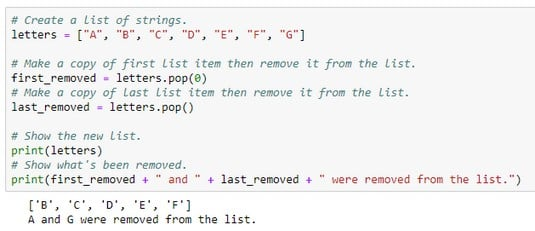Removing list items with pop().

Python also offers a `del` (short for delete) command that deletes any item from a list based on its index number (position). But again, you have to remember that the first item is zero. So, let's say you run the following code to delete item number 2 from the list:

```# Create a list of strings.
letters = ["A", "B", "C", "D", "E", "F", "G"]

# Remove item sub 2.
del letters

print(letters)```
Running that code shows the list again, as follows. The letter C has been deleted, which is the correct item to delete because letters are numbered 0, 1, 2, 3, and so forth.
`['A', 'B', 'D', 'E', 'F', 'G']`
You can also use del to delete an entire list. Just don’t use the square brackets and the index number. For example, the code you see below creates a list then deletes it. Trying to print the list after the deletion causes an error, because the list no longer exists when the `print()` statement is executed.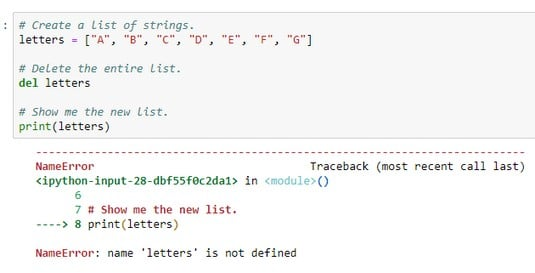Deleting a list and then trying to print it causes an error.

## Clearing out a Python list

If you want to delete the contents of a list but not the list itself, use `.clear()`. The list still exists; however, it contains no items. In other words, it's an empty list. The following code shows how you could test this. Running the code displays [] at the end, which lets you know the list is empty:
```# Create a list of strings.
letters = ["A", "B", "C", "D", "E", "F", "G"]

# Clear the list of all entries.
letters.clear()

# Show me the new list.
print(letters)
```
[]

## Counting how many times an item appears in a Python list

You can use the Python `count()` method to count how many times an item appears in a list. As with other list methods, the syntax is simple:
`<em>listname</em>.count(<em>x</em>)`
Replace listname with the name of your list, and x with the value you're looking for (or the name of a variable that contains that value).

The code in the image below counts how many times the letter B appears in the list, using a literal B inside the parentheses of `.count()`. This same code also counts the number of C grades, but that value was stored in a variable just to show the difference in syntax. Both counts worked, as you can see in the output of the program at the bottom. One was added to count the F's, not using any variables. The F’s were counted right in the code that displays the message. There are no F grades, so this returns zero, as you can see in the output.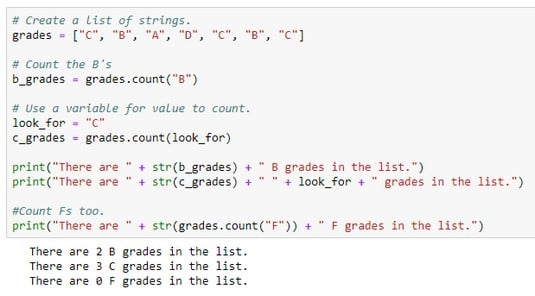Counting items in a list.

When trying to combine numbers and strings to form a message, remember you have to convert the numbers to strings using the `str()` function. Otherwise, you get an error that reads something like can only `concatenate str (not "int") to str`. In that message, `int` is short for integer, and `str` is short for string.

## Finding a Python list item's index

Python offers an .index() method that returns a number indicating the position, based on index number, of an item in a list. The syntax is:
`<em>listname</em>.index(<em>x</em>)`
As always, replace `listname` with name of the list you want to search. Replace x what whatever you're looking for (either as a literal or as a variable name, as always). Of course, there’s no guarantee that the item is in the list, and even if it is, there’s no guarantee that the item is in the list only once. If the item isn’t in the list, then an error occurs. If the item is in the list multiple times, then the index of the first matching item is returned.

The following image shows an example where the program crashes at the line `f_index = grades.index(look_for)` because there is no F in the list.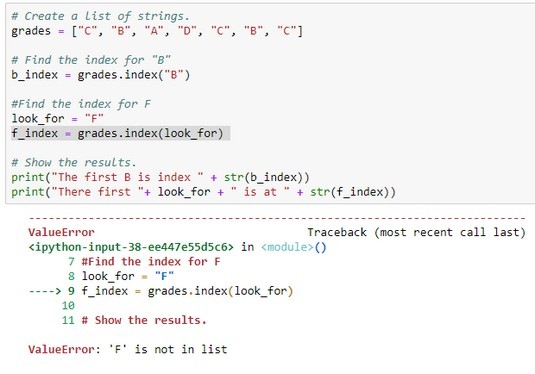Program fails when trying to find index of a nonexistent list item.

An easy way to get around that problem is to use an `if` statement to see whether an item is in the list before you try to get its index number. If the item isn't in the list, display a message saying so. Otherwise, get the index number and show it in a message. That code is as follows:

```# Create a list of strings.
grades = ["C", "B", "A", "D", "C", "B", "C"]
# Decide what to look for
look_for = "F"
# See if the item is in the list.
# If it's in the list, get and show the index.
print(str(look_for) + " is at index " + str(grades.index(look_for)))
else:
# If not in the list, don't even try for index number.
print(str(look_for) + " isn't in the list.")```

## Alphabetizing and sorting Python lists

Python offers a `sort()` method for sorting lists. In its simplest form, it alphabetizes the items in the list (if they’re strings). If the list contains numbers, they’re sorted smallest to largest. For a simple sort like that, just use `sort()` with empty parentheses:
`<em>listname</em>.sort()`
Replace `listname` with the name of your list. The following image shows an example using a list of strings and a list of numbers. In the example, a new list was created for each of them simply by assigning each sorted list to a new list name. Then the code prints the contents of each sorted list.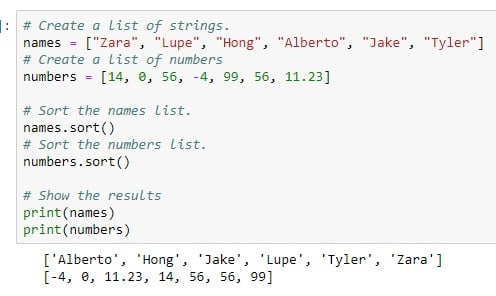Sorting strings and numbers.

If your list contains strings with a mixture of uppercase and lowercase letters, and if the results of the sort don't look right, try replacing `.sort()` with `.sort(key=lambda s:s.lower())` and then running the code again.

Dates are a little trickier because you can’t just type them in as strings, like `"12/31/2020"`. They have to be the `date` data type to sort correctly. This means using the `datetime` module and the `date()` method to define each date. You can add the dates to the list as you would any other list. For example, in the following line, the code creates a list of four dates, and the code is perfectly fine.

`dates = [dt.date(2020,12,31), dt.date(2019,1,31), dt.date(2018,2,28), dt.date(2020,1,1)]`
The computer certainly won't mind if you create the list this way. But if you want to make the code more readable to yourself or other developers, you may want to create and append each date, one at a time, so just so it’s a little easier to see what’s going on and so you don’t have to deal with so many commas in one line of code. The image below shows an example where an empty list named `datelist` was created:
`datelist = []`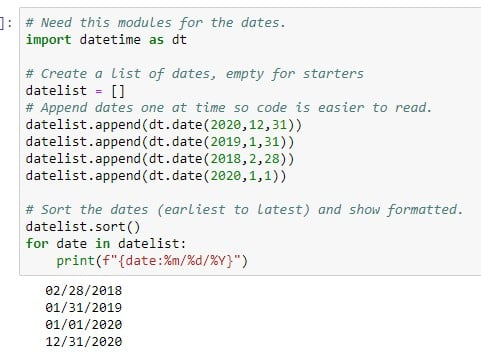Sorting and displaying dates in a nice format.

Then one date at a time was appended to the list using the `dt.date(<em>year</em>,<em>month</em>,<em>day</em>)` syntax.

After the list is created, the code uses `datelist.sort()` to sort them into chronological order (earliest to latest). You don’t need to use `print(datelist)` in that code because that method displays the dates with the data type information included, like this:

`[datetime.date(2018, 2, 28), datetime.date(2019, 1, 31), datetime.date (2020, 1, 1), datetime.date(2020, 12, 31)]`
Not the easiest list to read. So, rather than print the whole list with one `print()` statement, you can loop through each date in the list, and printed each one formatted with the f-string `%m/%d/%Y`. This displays each date on its own line in mm/dd/yyyy format, as you can see at the bottom of the image above.

If you want to sort items in reverse order, put `reverse=True` inside the sort() parentheses (and don't forget to make the first letter uppercase). The image below shows examples of sorting all three lists in descending (reverse) order using `reverse=True`.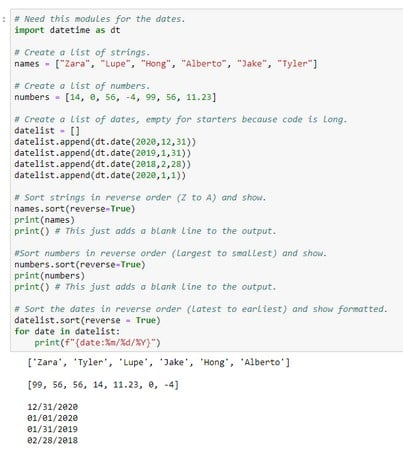Sorting strings, numbers, and dates in reverse order.

## Reversing a Python list

You can also reverse the order of items in a list using the `.reverse` method. This is not the same as sorting in reverse, because when you sort in reverse, you still actually sort: Z–A for strings, largest to smallest for numbers, latest to earliest for dates. When you reverse a list, you simply reverse the items in the list, no matter their order, without trying to sort them in any way.

The following code shows an example in which you reverse the order of the names in the list and then print the list. The output shows the list items reversed from their original order:

```# Create a list of strings.
names = ["Zara", "Lupe", "Hong", "Alberto", "Jake"]
# Reverse the list
names.reverse()
# Print the list
print(names)

['Jake', 'Alberto', 'Hong', 'Lupe', 'Zara']```

## Copying a Python list

If you ever need to work with a copy of a list, use the `.copy()` method so as not to alter the original list,. For example, the following code is similar to the preceding code, except that instead of reversing the order of the original list, you make a copy of the list and reverse that one. Printing the contents of each list shows how the first list is still in the original order whereas the second one is reversed:
```# Create a list of strings.
names = ["Zara", "Lupe", "Hong", "Alberto", "Jake"]

# Make a copy of the list
backward_names = names.copy()
# Reverse the copy
backward_names.reverse()

# Print the list
print(names)
print(backward_names)

['Zara', 'Lupe', 'Hong', 'Alberto', 'Jake']
['Jake', 'Alberto', 'Hong', 'Lupe', 'Zara']```
For future references, the following table summarizes the methods you've learned about.
 Method What it Does `append()` Adds an item to the end of the list. `clear()` Removes all items from the list, leaving it empty. `copy()` Makes a copy of a list. `count()` Counts how many times an element appears in a list. `extend()` Appends the items from one list to the end of another list. `index()` Returns the index number (position) of an element within a list. `insert()` Inserts an item into the list at a specific position. `pop()` Removes an element from the list, and provides a copy of that item that you can store in a variable. `remove()` Removes one item from the list. `reverse()` Reverses the order of items in the list. `sort()` Sorts the list in ascending order. Put `reverse=True` inside the parentheses to sort in descending order.